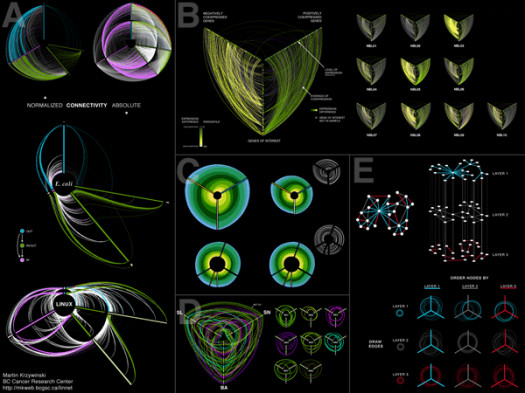# What Do You Know About Visualize Ratios?

10 Questions | Total Attempts: 113SettingsIn mathematics, a ratio is a relationship between two numbers indicating how many times the first number contains the second. For example, if a bowl of fruit contains eight oranges and six lemons, then the ratio of oranges to lemons is eight to six (that is, 8:6, which is equivalent to the ratio 4:3).

• 1.
Visualize Ratio is a topic under .....
• A.

Maths

• B.

Music

• C.

Bíology

• D.

Sociology

• 2.
Mathematics can be taught in .....
• A.

School

• B.

Home

• C.

Church

• D.

Mosque

• 3.
Visualize Ratios can be traced to be related to ....
• A.

Fractions

• B.

Ratio

• C.

Algebra

• D.

Decimals

• 4.
Who developed a theory of ratio and proportion as applied to numbers?
• A.

Eudoxus of Cnidus

• B.

Euclid

• C.

Socrates

• D.

The Pythagoreans

• 5.
The discovery of a theory of ratios that does not assume commensurability is probably due to who?
• A.

The Pythagoreans

• B.

Euclids

• C.

Eudoxus of Cnidus

• D.

Socrates

• 6.
Book V of Euclid's Elements has how many definitions, all of which relate to ratios?
• A.

18

• B.

20

• C.

12

• D.

10

• 7.
Visualize Ratios deals with visual representation of .....?
• A.

Decimals

• B.

Ratios

• C.

Fractions

• D.

Algebra

• 8.
In general, a comparison of the quantities of a two-entity ratio can be expressed as a .... derived from the ratio
• A.

Fraction

• B.

Decimal

• C.

Algebra

• D.

Square root

• 9.
Visualization of ratio is used to communicate .....?
• A.

Data

• B.

Calls

• C.

SMS

• D.

Fax

• 10.
..... was/is a scientist
• A.

Bill Clinton

• B.

Nelson Mandela

• C.

Bill Nye

• D.

Donald Trump

Related TopicsBack to top